# How to calculate average between two dates in Excel?

We can use Excel formulas to count, add values between two provided dates of a data range, and average numbers between two dates. I'll show you how to calculate the average of two dates in Excel.

The average of two dates can be calculated using the methods explained in this tutorial. Let’s understand step by step with an example.

### Step 1

In the first step, let’s assume we have a sample data. Please refer to the below screenshot for the same.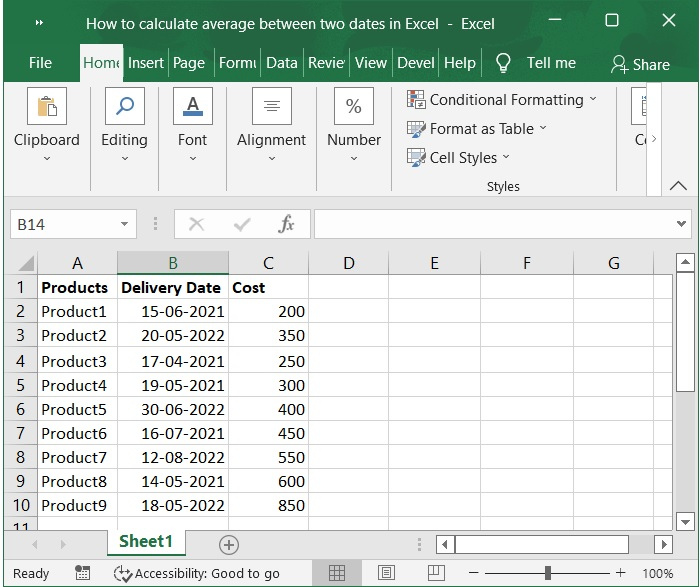### Step 2

Create a new cell where you can save the First Date, the Last Date, and the Average. Please check out screenshot below for the same.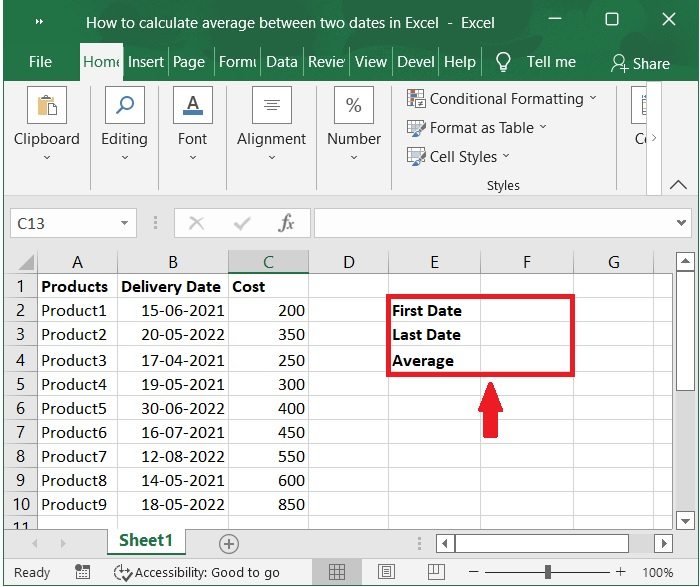### Step 3

Enter the First date of the time period whose average you want to calculate in the box labelled First Date. Put the date that the period you wish to count for the average ended in the box labelled Last Date. For instance, we count the average for the period between 13-05-2021 and 19-05-2022. Please refer to the screenshot below.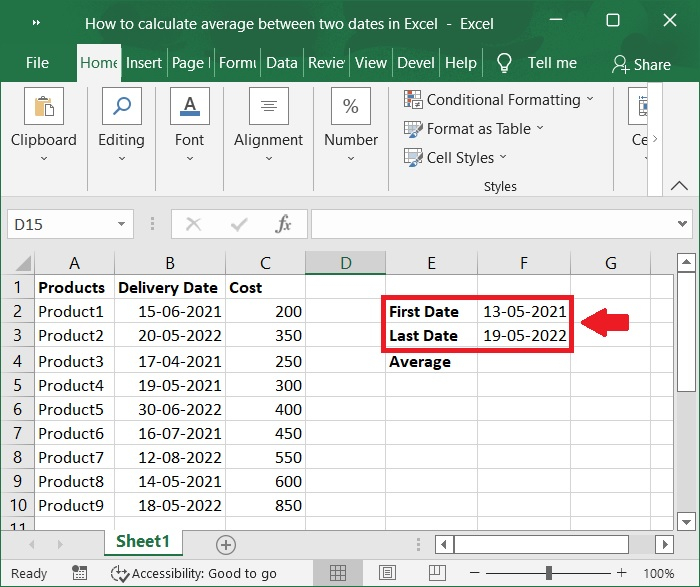### Step 4

Enter the formula into the column labelled Average. Check out screenshot below for the same.

### Formula

=AVERAGE(IF((B2:B10>=F2)*(B2:B10<=F3),C2:C10))


In the formula presented above, the given date range is represented by B2:B10, the First date is represented by F2, and the Last date is represented by F3. The Delivery Date is represented by B2:B10. C2:C10 is represented by cost.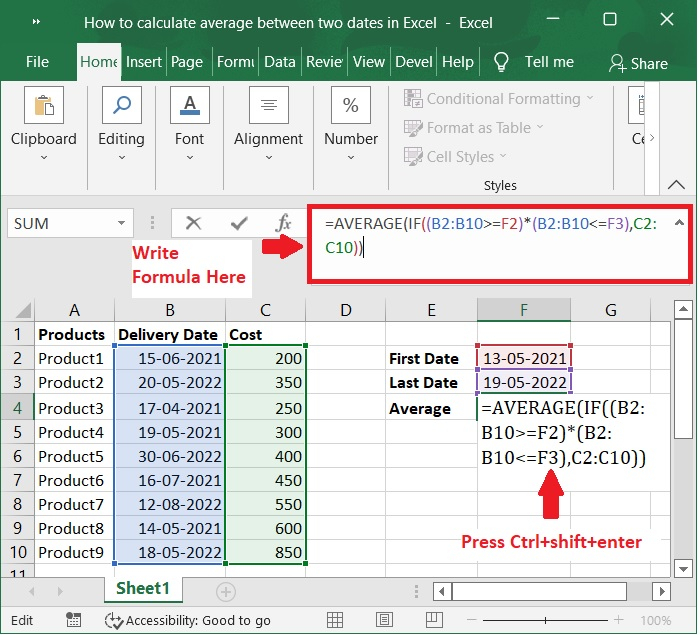### Step 5

To obtain the result after entering the formula, press Ctrl + Shift + Enter on your keyboard. Please refer to the below screenshot for the same.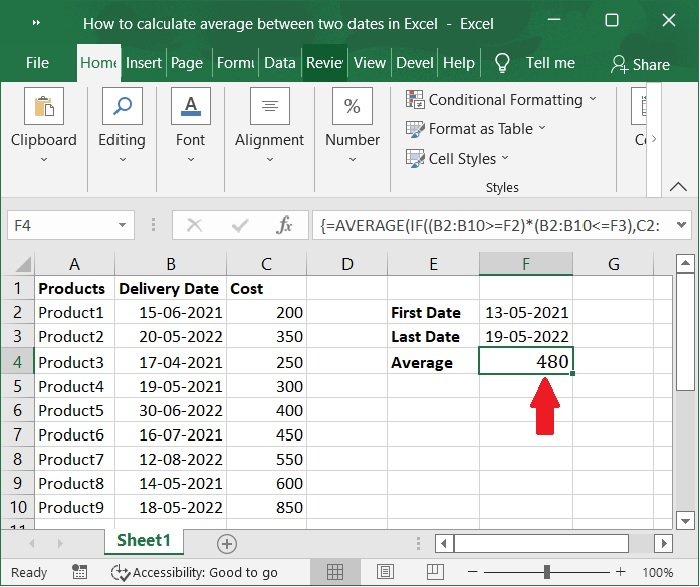### Step 6

In addition to the array formula that was just presented, I will now provide a standard formula, after which you should just press the Enter key. Please check out the screenshot below for the same.

### Formula

=SUMPRODUCT(--(B2:B10>=F2),--(B2:B10<=F3),C2:C10)/SUMPRODUCT(--(B2:B10>=F2),--(B2:B10<=F3))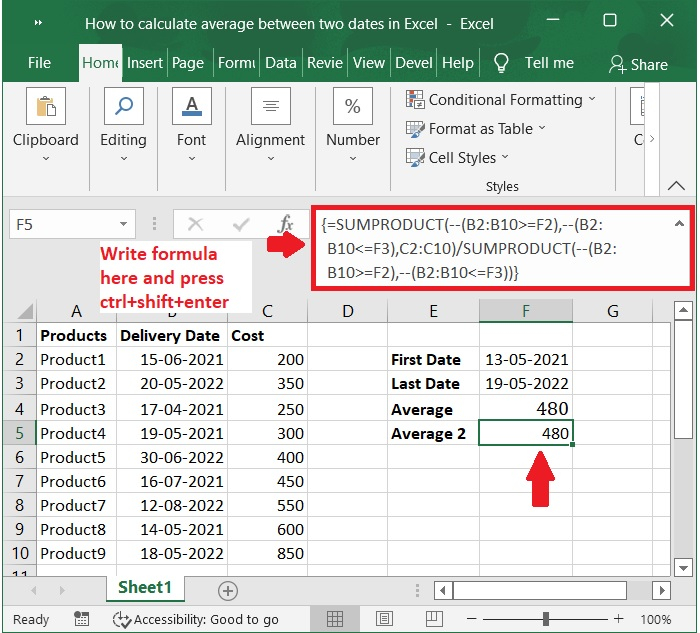## Conclusion

In this tutorial, we explained how to calculate average between two dates using formulas in Excel.